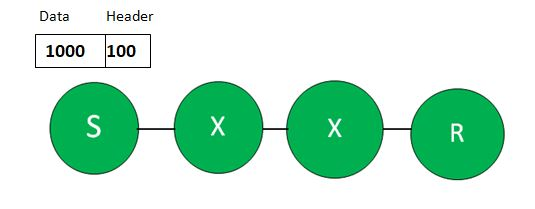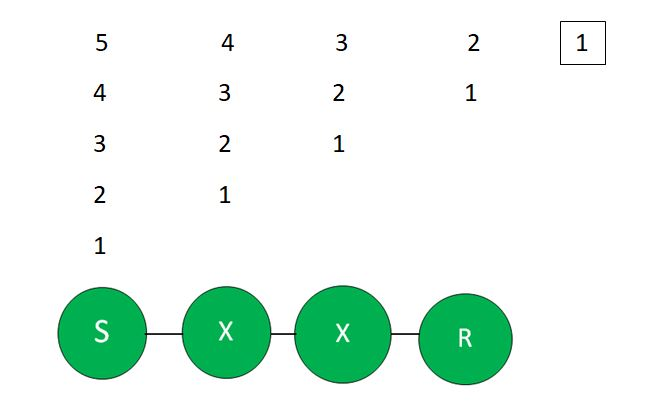# Pipelining in Packet Switching

Packet Switching:
A mode of data transmission in which a message is broken into a number of parts which are sent independently, over whatever route is optimum for each packet, and reassembled at the destination. Packet Switching uses Store and Forward technique while switching the packets; while forwarding the packet each hop first store that packet then forward.

As we know that the Data is divided into number of small packets to reduce the Total Delay. so, what do you think? Larger the number of packets, smaller the Delay?
No, Actually this is not True. Okay let’s understand with the example.

Example:

```Given,

Data size = 1000 Bytes
Bandwidth = 1 MBps
Number of Hops = 3
assume Propagation dalay = 0
```

Explanation: These are different scenario as following below.

Case-1: If the number of Packets = 1```Size of the Data Packet,
= Data size + Header size
= 1000 + 100 = 1100 Bytes

Transmission delay
= L/BW = 1100/10^6 = 1.1 ms

Total time taken
= number of Hopes * Transmission delay
= 3 * 1.1 = 3.3 ms ```

Case-2: If the number of Packets = 5

```Size of each Data Packet
= (1000/5) + 100 = 300 Bytes

Transmission Delay for each Packet
= 300/10^6 = 0.3 ms ``````Time taken by 1st Packet,
= No of Hops * Transmission delay
= 3*0.3 = 0.9 ms

Time taken by the remaining 4 Packets
= 4*Transmission delay
= 4*0.3 = 1.2 ms

Total Time Taken,
= 0.9+1.2 = 2.1 ms ```

Case-3: If the no of Packets = 10

```Size of each Data Packet
= (1000/10) + 100 = 200 Bytes

Transmission delay for each packet
= 200/10^6 = 0.2 ms

Time Taken by first Packet
= 3*0.2 = 0.6 ms

Time Taken by remaining Packets
= 9*0.2 = 1.8ms

Total Time Taken
= 0.6+1.8 = 2.4 ms ```

Case-4: If the no of Packets = 20

```Size of each Data Packet
= (1000/20) + 100 = 150 Bytes

Transmission delay for each Packet
= 150/10^6 = 0.15 ms

Time Taken by 1st Packet
= 3*0.15 = 0.45 ms

Time Taken by remaining 19 Packets
= 19*0.15 = 2.85 ms

Total Time Taken
= 0.45 + 2.85 = 3.3 ms ```

As we can see that there exist a threshold value at which the Total time decreases and if we increase the number of Packets after that limit, Total time starts increasing. Initially the time is decreasing for some limit and after that time is going to increase. If the number of Packets are very large then it may take time more than the time taken by the transmission of single packet.My Personal Notes arrow_drop_upCheck out this Author's contributed articles.

If you like GeeksforGeeks and would like to contribute, you can also write an article using contribute.geeksforgeeks.org or mail your article to contribute@geeksforgeeks.org. See your article appearing on the GeeksforGeeks main page and help other Geeks.

Please Improve this article if you find anything incorrect by clicking on the "Improve Article" button below.

Article Tags :
Practice Tags :

1

Please write to us at contribute@geeksforgeeks.org to report any issue with the above content.